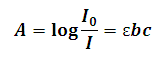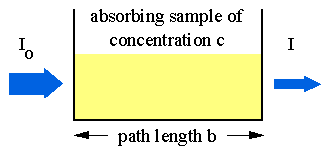# Beer Lambert Law Calculator

This Calctown Calculator calculates the Absorbance and Molar absorptivity of a given solution using the Beer-Lambert Law.

W/m2
W/m2
cm
mol/dm3

#### Result

m2/molwhere

A = absorbance

I = intensity of light after passing through the solution

I0 = intensity of light before passing through the solution

ε = molar absorptivity of the solution

b = path length in m

c = concentration of solution in mol m-3